# Frank solutions for Class 9 Physics ICSE chapter 2 - Motion in One Dimension [Latest edition]

#### Chapters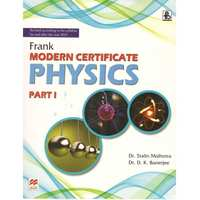## Chapter 2: Motion in One Dimension

Exercise
Exercise [Pages 61 - 62]

### Frank solutions for Class 9 Physics ICSE Chapter 2 Motion in One Dimension Exercise [Pages 61 - 62]

Exercise | Q 1 | Page 61

Define the state of rest.

Exercise | Q 2 | Page 61

What do you mean by a vector quantity?

Exercise | Q 4 | Page 61

How is a vector represented?

Exercise | Q 5 | Page 61

A book is lying in an almirah. Is it at rest or in motion?

Exercise | Q 6 | Page 61

What do you mean by motion?

Exercise | Q 7 | Page 61

Are rest and motion relative?

Exercise | Q 8 | Page 61

Out of force and energy, which one is vector?

Exercise | Q 9 | Page 61

Give two examples of scalars.

Exercise | Q 10 | Page 61

Different positions of a body are given below. Which of the two positions lie on the same straight line?
(i) 8 m, 60o NE
(ii) 3 m, 60o NE
(iii) 5 m, 60o EN
(iv) 5 m, 60o NW

Exercise | Q 11 | Page 61

A quantity can be represented only when its magnitude and direction are specified. Is it scalar or vector?

Exercise | Q 12 | Page 62

Are the passengers sitting in a train in motion or at rest with respect to each other?

Exercise | Q 13 | Page 62

You are at rest as well as in motion. Justify the statement giving your point of view.

Exercise | Q 14 | Page 62

A train is moving out of a railway station. Is the platform at rest or in motion with respect to the moving train? Justify your answer.

Exercise | Q 15 | Page 62

Represent the location of an object described as at 15 m, 45o west of north, on a graph paper.

Exercise | Q 16 | Page 62

Represent the position of a body described as at 15 m, 30o north of west, on a graph paper.

Exercise | Q 17 | Page 62

Name the physical quantity representing the magnitude and its direction.

Exercise | Q 18.1 | Page 62

Exercise | Q 18.2 | Page 62

Exercise | Q 18.3 | Page 62

Can we multiply two scalars?

Exercise | Q 18.4 | Page 62

Can we add a scalar quantity to a vector quantity?

Exercise | Q 18.5 | Page 62

Can we subtract two scalars?

Exercise | Q 18.6 | Page 62

Can we subtract a scalar quantity from a vector quantity? Is the reverse true?

Exercise | Q 18.7 | Page 62

Can we multiply vectors?

Exercise | Q 19 | Page 62

What do you mean by the distance traveled by a body?

Exercise | Q 20 | Page 62

Can distance covered by a body be less than the magnitude of its displacement?

Exercise | Q 21 | Page 62

Define Displacement.

Exercise | Q 21 | Page 62

Define displacement.

Exercise | Q 22 | Page 62

Write the SI units for measurement of distance and displacement.

Exercise | Q 23 | Page 62

Show the difference between distance and displacement with the help of a diagram.

Exercise | Q 24 | Page 62

Can a body have negative displacement?

Exercise | Q 25 | Page 62

Under what condition is the displacement of a body equal to the distance traveled by it?

Exercise | Q 26 | Page 62

Write two differences between distance and displacement.

Exercise | Q 27.1 | Page 62

Calculate the distance and displacement in the following case: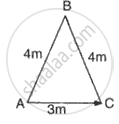Exercise | Q 27.2 | Page 62

Calculate the distance and displacement in the following case: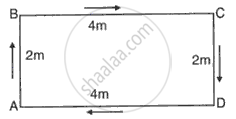Exercise | Q 27.3 | Page 62

Calculate the distance and displacement in the following case: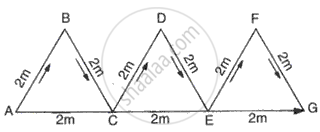Exercise | Q 28.1 | Page 62

A body is moving along a circular path of radius r. What will be the distance and displacement of the body when it completes:
Half a revolution

Exercise | Q 28.2 | Page 62

A body is moving along a circular path of radius r. What will be the distance and displacement of the body when it completes:
(3/4) th of a revolution

Exercise | Q 28.3 | Page 62

A body is moving along a circular path of radius r. What will be the distance and displacement of the body when it completes:
Full revolution

Exercise | Q 28.4 | Page 62

A body is moving along a circular path of radius r. What will be the distance and displacement of the body when it completes:
Two full revolutions

Exercise | Q 29.1 | Page 62

Ashok starts from his house and travels 3 km to reach his school. On finding the school gates closed, he returns to his house. What is?
The distance traveled by Ashok.

Exercise | Q 29.2 | Page 62

Ashok starts from his house and travels 3 km to reach his school. On finding the school gates closed, he returns to his house. What is?
The displacement of Ashok

Exercise | Q 30 | Page 62

A satellite makes a complete round along its circular orbit. What is its displacement?

Exercise | Q 31.1 | Page 62

An object is thrown vertically upwards. It rises upto a height H and then returns to its initial position. Calculate
The total distance traveled by the object

Exercise | Q 31.2 | Page 62

An object is thrown vertically upwards. It rises upto a height H and then returns to its initial position. Calculate
The displacement of the object.

Exercise [Pages 79 - 80]

### Frank solutions for Class 9 Physics ICSE Chapter 2 Motion in One Dimension Exercise [Pages 79 - 80]

Exercise | Q 1 | Page 79

Define speed of a body.

Exercise | Q 2 | Page 79

What do you understand by the average speed of a body?

Exercise | Q 4 | Page 79

Do speed and average speed of a body have the same meaning?

Exercise | Q 5 | Page 79

Which is greater?
(i) 60 km/hr
(ii) 15 m/s

Exercise | Q 6 | Page 79

Convert 20 m/s into km/hr.

Exercise | Q 7 | Page 79

Which is greater?
(i) 36 km/hr
(ii) 8.5 m/s

Exercise | Q 8.9 | Page 79

What are the SI units of velocity?

Exercise | Q 10 | Page 79

Arrange the following speeds in the increasing order:
(i) A bicycle moving with a speed of 36 km/h.
(ii) A car moving with a speed of 2 km/min.
(iii) An athlete running with a speed of 7 m/s.

Exercise | Q 11 | Page 79

A boy covers half of his journey with a uniform speed of u and the other half with a uniform speed of v. What is the average speed for the whole journey?

Exercise | Q 12 | Page 79

Does uniform speed mean constant speed?

Exercise | Q 13 | Page 79

A body goes from P to Q with a uniform speed of u and immediately returns back to P at a uniform speed of v. What is the average speed for the whole journey?

Exercise | Q 14 | Page 79

A body goes from P to Q with a uniform speed of u and immediately returns back to P at a uniform speed of v. what is the average velocity for the whole journey?

Exercise | Q 15 | Page 79

A car travels from P to Q at a uniform speed of 20 ms-1 and immediately turns back and travels towards Q with a uniform speed of 30 ms-1. What is the average speed for the whole journey?

Exercise | Q 16 | Page 79

How does speed differ from velocity?

Exercise | Q 17 | Page 79

When do the speed and velocity of a moving body become equal?

Exercise | Q 18 | Page 79

What is meant by a constant velocity? Is it same as uniform velocity?

Exercise | Q 19 | Page 79

Define acceleration.

Exercise | Q 20 | Page 79

What is the SI unit of acceleration?

Exercise | Q 21 | Page 80

The acceleration of a moving body is constant in magnitude and direction. Must the path of the body be a straight line?
If not, given an example.

Exercise | Q 22 | Page 80

The relation S= v x t can easily be used to find the tota l distance covered by a body moving with non -uni form speed.  (Yes/ no)

Exercise | Q 23 | Page 80

The area under a speed-time graph in a given intervals gives the total distance covered by a body irrespective of its motion being uniform or non-uniform.

Exercise | Q 24 | Page 80

The area of the right triangle under a speed-time graph is 500 m, in a time interval of 20 s. What is the speed of the body? Is the motion uniform or non-uniform?

Exercise | Q 25 | Page 80

What does a positive acceleration mean?

Exercise | Q 26 | Page 80
What does a negative acceleration mean?
Exercise | Q 27 | Page 80

A body falls towards the earth. Does it have positive or negative acceleration?

Exercise | Q 28 | Page 80

The acceleration of a body is 8.5 ms-2. What does this statement mean?

Exercise | Q 29 | Page 80

Write the SI unit of retardation.

Exercise | Q 30 | Page 80

A car traveling at 60 km/h, stops on applying brakes in 10 seconds. What is its acceleration?

Exercise | Q 31 | Page 80

A quantity is measured to be -30 m/s. Is it speed or velocity?

Exercise | Q 32 | Page 80

Give the name of the physical quantity that corresponds to the rate of change of displacement.

Exercise | Q 33 | Page 80

Can the speed of a body be negative?

Exercise | Q 34 | Page 80

What kind of a velocity does a flying bird most likely to have?
(a) Uniform velocity, or
(b) Non- uniform velocity.

Exercise | Q 35 | Page 80

Find the initial velocity of a car which is stopped in 10 seconds by applying brakes. Retardation due to brakes is 2.5 ms-2.

Exercise | Q 36 | Page 80

What do you understand by the equations of motion?

Exercise | Q 36 | Page 80

What do you understand by the equations of motion?

Exercise | Q 37 | Page 80

Write down the three equations of motion.

Exercise | Q 38 | Page 80

How many variables are present in each equation of motion?

Exercise | Q 39 | Page 80

What are the uses of equations of motion?

Exercise | Q 40 | Page 80

Write the SI unit of acceleration and retardation.

Exercise | Q 41 | Page 80

Name the physical quantity which is equal to the area under speed-time graph.

Exercise | Q 42 | Page 80

Distinguish between uniformly and non-uniformly accelerated motions.

Exercise | Q 43 | Page 80

Can you use the relation [B1]?

Exercise | Q 44 | Page 80

How does the slope of a speed-time graph give the acceleration of a body moving along a straight line?

Exercise | Q 45 | Page 80

Give two examples of uniform circular motion from your daily life.

Exercise | Q 46 | Page 80

How will you use a speed-time graph to find whether the acceleration of the body is uniform or not?

Exercise | Q 47 | Page 80

The graph of one quantity versus another result in a straight line. How are the quantities related to each other?

Exercise | Q 48 | Page 80

A car travels at a uniform speed of 42 km/h. In 10 minutes, how much distance would it cover?

Exercise | Q 49 | Page 80

The initial velocity of a car is 10 ms-1. It moves with an acceleration of 2 ms-2. What will be its speed after 10 seconds?

Exercise | Q 50 | Page 80

The speed of a car increases from 10 km/h to 64 km/h in 10 seconds. What will be its acceleration?

Exercise | Q 51 | Page 80

Given on th e side are a few speed - time graphs for various objects moving along a stra ight line. Refer below figure. (a), (b), (c) and (d).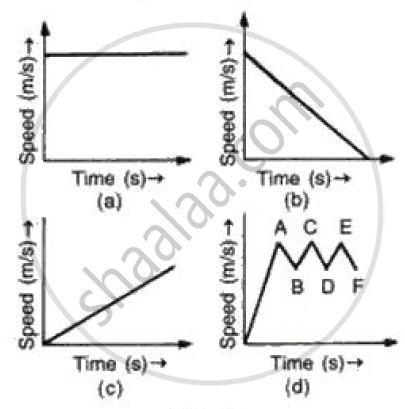Which of these graphs represent
(a) Uni form motion
(b) Motion with speed increasing
(c) Motion with speed decreasing and
(d) Motion with speed oscillating.?

Exercise | Q 52 | Page 80

Can a body have negative speed?

Exercise | Q 53 | Page 80

Is distance covered during nth second more than the distance covered in n seconds?

Exercise | Q 54 | Page 80

Draw the speed-time graph of a body when its initial speed is not zero and the speed increases uniformly with time.

Exercise | Q 55 | Page 80

Draw the speed-time graph of a body starting from some point P, gradually picking up speed, then running at a uniform speed and finally slowing down to stop at some point Q.

Exercise | Q 56 | Page 80

What can you conclude if the speed-time graph of a body is a curve moving upwards starting from the origin?

Exercise | Q 57 | Page 80

What can you conclude if the speed-time graph of a body is a straight line sloping upwards and not passing through the origin?

Exercise | Q 58.1 | Page 80

Draw the following graph:
Speed versus time for a stepped motion

Exercise | Q 58.2 | Page 80

Draw the following graph:
Speed versus time for a non-uniform acceleration.

Exercise | Q 58.3 | Page 80

Draw the following graph:
Distance versus time for a body at rest.

Exercise | Q 58.4 | Page 80

Draw the following graph:
Speed versus time for a fluctuating speed.

Exercise | Q 58.5 | Page 80

Draw the following graph:
Speed versus time for a uniformly retarded motion.

Exercise | Q 59.1 | Page 80

Interpret the following graph:Exercise | Q 59.2 | Page 80

Interpret the following graph: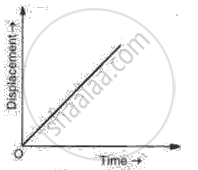Exercise | Q 60 | Page 80

Given below are the speed -time graphs. Match them with their corresponding motions :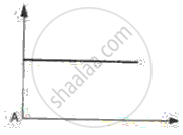(a) Uniformity retared motion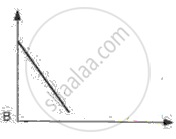(b) Non-uniformity acceleration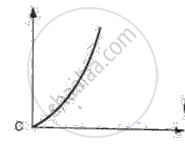(c) Non-uniform motion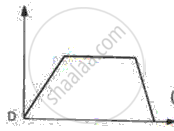(d) uniform motion
Exercise | Q 61 | Page 80

Derive the equations
(i) v= u+at and
(ii) v2-u2= 2as, where the symbols have their usual meanings.

Exercise | Q 62 | Page 80

Derive th e equation of motion.
S = ut+ 1/2 at2,
Where the symbols have their usual meanings

Exercise [Pages 83 - 87]

### Frank solutions for Class 9 Physics ICSE Chapter 2 Motion in One Dimension Exercise [Pages 83 - 87]

Exercise | Q 1 | Page 83

Write two examples of vectors.

Exercise | Q 2 | Page 83

Write the SI unit of retardation.

Exercise | Q 3 | Page 83

Name the physical quantity associated with the rate of change of displacement with time.

Exercise | Q 4 | Page 83

Define angular velocity

Exercise | Q 5 | Page 83
How many types of rectilinear motion are there?
Exercise | Q 6 | Page 83

When a body said to have a uniform velocity?

Exercise | Q 7 | Page 83

Out of energy and acceleration, which one is a vector?

Exercise | Q 8 | Page 83

What does the slope of speed-time graph indicate?

Exercise | Q 9 | Page 83

A stone is dropped from a certain height. Is its motion uniform or non-uniform?

Exercise | Q 10 | Page 83

The distance covered by a body is directly proportional to the square of the time elapsed. What can you say about its acceleration?

Exercise | Q 11 | Page 83

Which of the following graphs represents a motion with negative acceleration?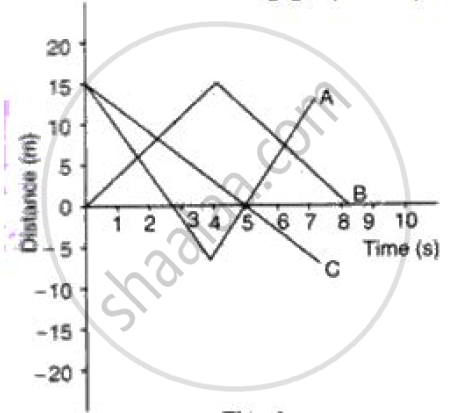Exercise | Q 12.1 | Page 83

State if the following situation is possible:
A body moving with constant acceleration but with Zero velocity.

Exercise | Q 12.2 | Page 83

State if the following situation is possible:
A body moving horizontally with an acceleration in vertical direction.

Exercise | Q 12.3 | Page 83

State if the following situation is possible:
A body moving with a constant velocity in an accelerated motion.

Exercise | Q 13 | Page 83

Figure shows displacement- time graph of two objects A and B moving in a stra ight line. Which object is moving fa ster?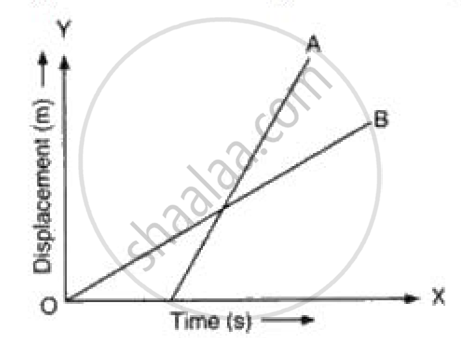Exercise | Q 14.1 | Page 83

What can you say about the nature of motion of a body of its displacement-time graph is:
A straight line parallel to line axis?

Exercise | Q 14.2 | Page 83

What can you say about the nature of motion of a body of its displacement-time graph is:
A straight line inclined to the time axis with an acute angle?

Exercise | Q 15 | Page 83

Is it possible for an accelerating body to have constant speed?

Exercise | Q 16.1 | Page 83
What can you say about the motion of a body if:
Its time-displacement graph is a straight line.
Exercise | Q 16.2 | Page 83

What can you say about the motion of a body if:
Its time-velocity graph is a straight line

Exercise | Q 17 | Page 83

Is the average speed during different time intervals for a uniform motion the same or different?

Exercise | Q 18 | Page 83

What is the velocity of a stone thrown vertically upwards at its maximum height?

Exercise | Q 19 | Page 83

Why does the velocity of a stone thrown vertically upwards decreases?

Exercise | Q 20 | Page 83

What is the relation between linear velocity and linear speed?

Exercise | Q 21.1 | Page 83

Draw distance-time graph to show:
Increasing velocity

Exercise | Q 21.2 | Page 83

Draw distance-time graph to show:
Uniform velocity

Exercise | Q 21.3 | Page 83

Draw distance-time graph to show:
Decreasing velocity

Exercise | Q 22.1 | Page 83

Draw velocity-time graph to show:
Acceleration
Write a sentence to explain the shape of graph.

Exercise | Q 22.2 | Page 83

Draw velocity-time graph to show:
Deceleration
Write a sentence to explain the shape of graph.

Exercise | Q 22.3 | Page 83

Draw velocity-time graph to show:
Zero acceleration
Write a sentence to explain the shape of graph.

Exercise | Q 23.1 | Page 84

Interpret the following graph: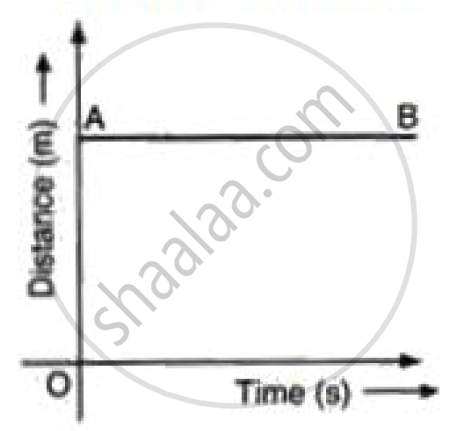Exercise | Q 23.3 | Page 84

Interpret the following graph: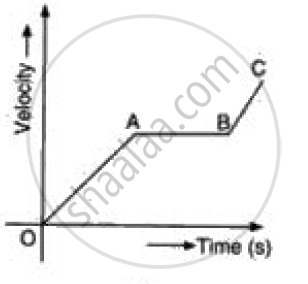Exercise | Q 24.1 | Page 84

During circular motion, which physical quantity of the body:
Remains constant

Exercise | Q 24.2 | Page 84

During circular motion, which physical quantity of the body:
Changes continuously

Exercise | Q 25 | Page 84

Is the following statement correct? If not, modify it suitably to make it correct. "The earth moves round the sun with uniform velocity".

Exercise | Q 26 | Page 84

A particle moves along a circular path. How many times does it change its direction in two complete rounds?

Exercise | Q 27 | Page 83

A body moves along a circular path of radius r. When it completes three complete rounds, what is the ratio of distance covered to its displacement?

Exercise | Q 28 | Page 84

What happens to the stepwise increasing speed-time graph when the size of each step is made very small?

Exercise | Q 29 | Page 84

A body has an acceleration of -3.5 ms-2. What is its retardation?

Exercise | Q 30 | Page 84

The average time taken by a normal person to react to an emergency is one fifteenth of a second and is called the 'reaction time'. If a bus is moving with a velocity of 60 km/h and its driver sees a child running across the road, how much distance would the bus had moved before he could press the brakes? The reaction time of the people increases when they are intoxicated. How much distance had the bus moved if the reaction time of the driver were 1/2 s under the influence of alcohol?

Exercise | Q 31 | Page 84

A girl had designed a clap switch for a science exhibition that enabled her to switch on or off an alarm just with clapping of hands. While testing her device in a hall, she noticed that once the alarm has sounded it followed with another one due to echo of the clap, that is, the sound reflected by the walls. She recorded the two soundings of alarm with her tape recorder and found out that time difference in between them is 0.1 s. If the distance of the walls be 15 m, calculate the speed of sound.

Exercise | Q 32 | Page 84

An artificial satellite is moving in a circular orbit of radius nearly 42,250 km. Calculate its linear velocity, if it takes 24 hours to revolve round the earth

Exercise | Q 33 | Page 84

A circular cycle track has a circumstance of 314 m with AB as one of its diameter. A cyclist travels from A to B along the circular path with a velocity of constant magnitude 15. 7 m/ s. Find :
(a) The distance moved by the cyclist.
(b) The displacement of the cyclist if AB represents north-south direction .
(c) The average velocity of the cyclist.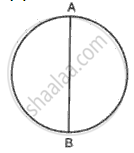Exercise | Q 34 | Page 84

What does the slope of velocity-time graph represent?

Exercise | Q 34 | Page 84

What does the slope of velocity-time graph represent?

Exercise | Q 35 | Page 84

Figure shows the velocity-time graphs for two objects A and B moving in same direction . Which object has the greater a cceleration?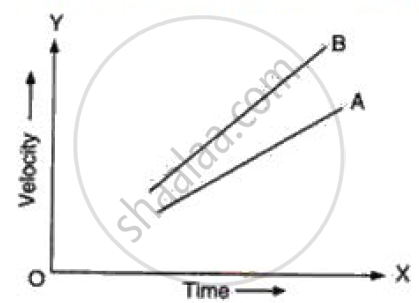Exercise | Q 36.1 | Page 85

Sketch the shape of the velocity-time graph for a body moving with:
Uniformly velocity

Exercise | Q 36.2 | Page 85

Sketch the shape of the velocity-time graph for a body moving with:
Uniform acceleration.

Exercise | Q 37 | Page 83

Derive the equation
S = ut+ 1/2 at2
Using a speed- time graph

Exercise | Q 38 | Page 85

Derive the equation
V2-u2 = 2as

Exercise | Q 39 | Page 85

For a body moving along a circular path, show that:
Linear speed = Angular velocity X radius of the circle

Exercise | Q 40 | Page 85

Draw the distance-time graphs of the bodies P and Q starting from rest, moving with uniform speeds with P moving faster than Q.

Exercise | Q 41 | Page 85

A body covers half the distance with the speed A and the other half with speed B. What will be the average speed for the whole journey?

Exercise | Q 42 | Page 85

A car travels 30 km at a uniform speed of 60 km/h and the next 30 km at a uniform speed of 20 km/h. What is its average speed?

Exercise | Q 43 | Page 85

On a 120 km track, a train travels the first 40 km at a uniform speed of 30 km/h. How fast must the train travel the next 80 km, so as to average 60 km/h for the entire trip.

Exercise | Q 44.1 | Page 85

Figure shows the distance-time graph of three students A, B and C. On the basis of the graph, answer the following :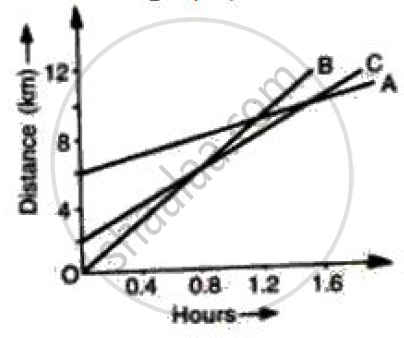Which of the three is traveling the fastest?

Exercise | Q 44.2 | Page 85

Figure shows the distance-time graph of three students A, B and C. On the basis of the graph, answer the following :Will the three ever meet at any point on the road?

Exercise | Q 44.3 | Page 85

Figure shows the distance-time graph of three students A, B and C. On the basis of the graph, answer the following :When B meets A, where is C?

Exercise | Q 44.4 | Page 85

Figure shows the distance-time graph of three students A, B and C. On the basis of the graph, answer the following :How far did B travel between the time he passed C and A?

Exercise | Q 45 | Page 85

In figure the position of a body times is shown . Calculate the speed of the body as it moves from 0 to A, A to B, B to C and i ts average speed .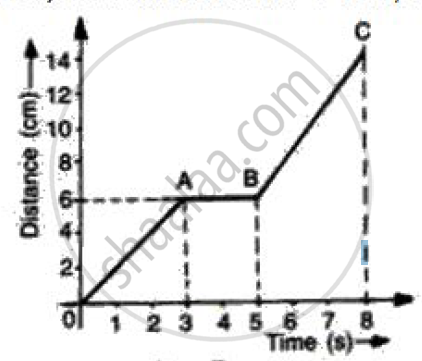Exercise | Q 46 | Page 85

Two friends leave Delhi for Chandigarh in their cars. A starts at 5 am and moves with a constant speed of 30 km/h, whereas B starts at 6 am and moves with a constant speed of 40 kmh-1. Plot the distance-time graph for their motion and find at what time the two friends will meet and at what distance from Delhi.

Exercise | Q 47 | Page 85

A car accelerates uniformly from a velocity of 18 km/h to 36 km/h in 15 min. What is its acceleration?

Exercise | Q 48 | Page 85

A car is moving with a speed of 50 km/h. One second later, its speed is 55 km/h. What is its acceleration?

Exercise | Q 49.1 | Page 85

Convert the following acceleration:
7200 km/h2 into m/s2

Exercise | Q 49.2 | Page 85

Convert the following acceleration:
1/36 m/s2 into km/h2

Exercise | Q 50 | Page 85

A body moves along a straight road with a speed of 20 m/s and has a uniform acceleration of 5 m/s2. What will be its speed after 2 s?

Exercise | Q 51 | Page 85

A car moving with a uniform acceleration of 10 m/s2 on a straight road changes its speed from 10 m/s to 30 m/s. Find the time lapsed for the change of speed.

Exercise | Q 52.1 | Page 86

The graph shows how the velocity of a scooter varies with time in 50 s. Interpret the graph.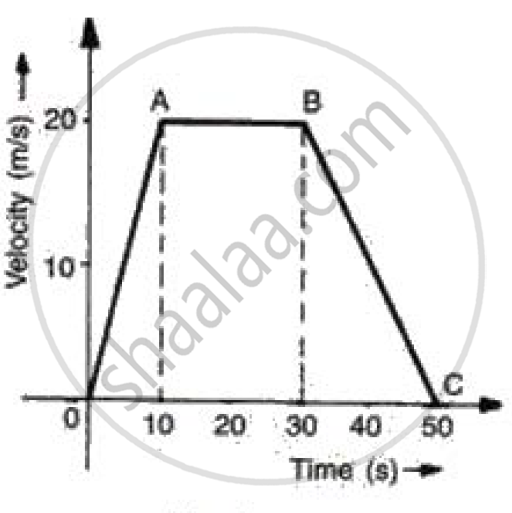Exercise | Q 52.2 | Page 86

The graph shows how the velocity of a scooter varies with time in 50 s.
Work out: Acceleration.Exercise | Q 52.2 | Page 86

The graph shows how the velocity of a scooter varies with time in 50 s.
Work out: The distance traveled in 10 s, 20 s, and 50 s.Exercise | Q 52.3 | Page 86

The graph shows how the velocity of a scooter varies with time in 50 s.
Work out: DecelerationExercise | Q 53.1 | Page 86

A car accelerates to a velocity of 30 m/s in 10 s and then decelerates for 20 s so that it stops. Draw a velocity-time graph to represent the motion and find:
The acceleration.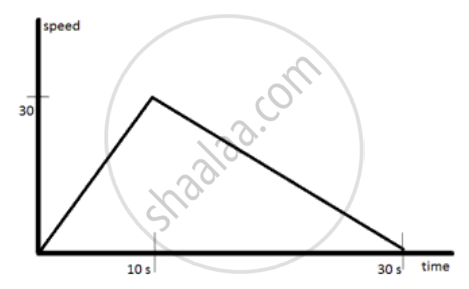Exercise | Q 53.2 | Page 86

A car accelerates to a velocity of 30 m/s in 10 s and then decelerates for 20 s so that it stops. Draw a velocity-time graph to represent the motion and find:
The Deceleration.Exercise | Q 53.3 | Page 86

A car accelerates to a velocity of 30 m/s in 10 s and then decelerates for 20 s so that it stops. Draw a velocity-time graph to represent the motion and find:
Distance travelledExercise | Q 54 | Page 86

A body moves along a straight track. Half of the distance it covers with a velocity of 40 ms-1 and the remaining half with a velocity of 60 ms-1. What is the average velocity for the whole journey?

Exercise | Q 55 | Page 86

A body goes from A to B with a velocity of 30 ms-1 and comes back from B to A with a velocity of 20 ms-1. What is the average velocity of the body for the whole journey?

Exercise | Q 56 | Page 86

A body starts to slide over a horizontal surface with an initial velocity of 0.5 ms-1. Due to friction, its velocity decreases at the rate of 0.05 ms-2. How much time will it take for the body to stop?

Exercise | Q 57 | Page 86

A train is moving at a speed of 90 km/h. On applying brakes, a retardation of 2.5 ms-2 is created. At what distance before, should the driver apply the brakes to stop the train at the station?

Exercise | Q 58 | Page 86

A train travels the first 30km of its journey at 30km/h. Find the speed it should travel the next 90km to have an average speed of 60km/h.

Exercise | Q 59 | Page 86

A 50 m long train passes over a bridge at a speed of 30 km/h. If it takes 36 seconds to cross the bridge, calculate the length of the bridge.

Exercise | Q 60 | Page 86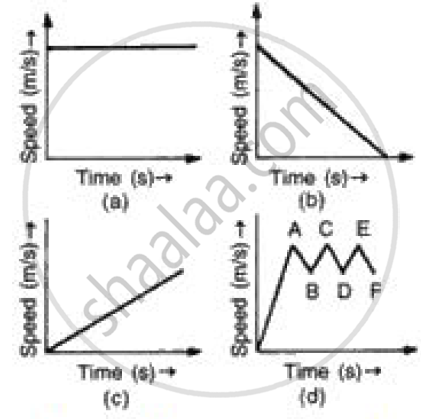(i) In speed time graph uniform motion is given by a straight line parallel to x axis so figure (a) denotes the uniform motion.
(ii) In speed time graph motion with increasing speed is shown by straight line with positive slope so figure (c) denotes the motion with speed increasing.
(iii) In speed time graph motion with decreasing speed is shown by straight line with negative slope so figure (b) denotes the motion with speed decreasing.
(iv) In speed time graph motion with oscillating speed is shown by zigzag line so figure {d) denotes the motion with speed oscillating.

Exercise | Q 61.1 | Page 86

Figure represents graphically the velocity of a car moving along a straight road over a period of 100 hours.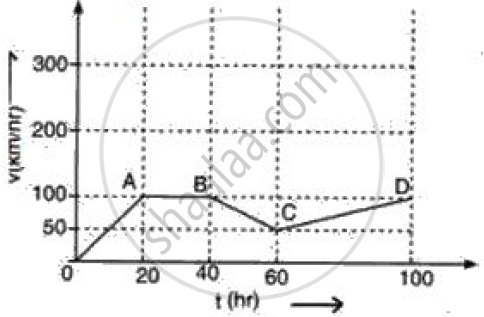How long is the body travelling with a uniform velocity?

Exercise | Q 61.2 | Page 86

Figure represents graphically the velocity of a car moving along a straight road over a period of 100 hours.Calculate the acceleration along AB and the retardation along BC.

Exercise | Q 61.3 | Page 86

The figure represents graphically the velocity of a car moving along a straight road over a period of 100 hours.Calculate the distance travelled in the last 40 h.

Exercise | Q 62 | Page 87

Write down the type of motion of a body in each of the following distance time-graph.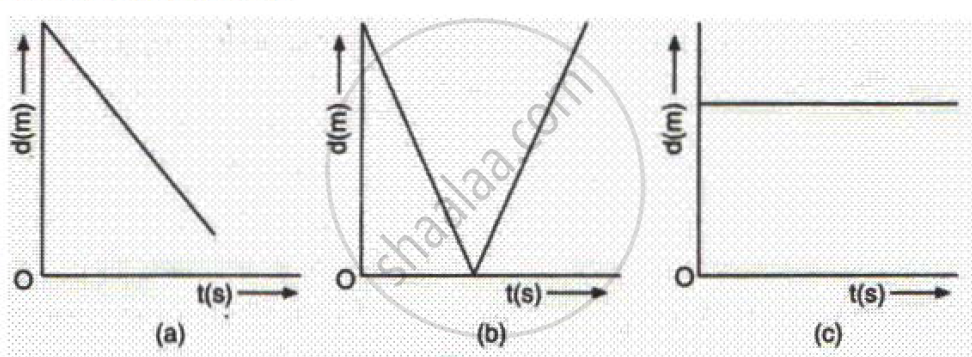Exercise | Q 63.1 | Page 87

The table below shows the distance travelled by two vehicles A and B during each second:

 Time (s) 0 1 2 3 4 5 6 7 Distance travelled by A (m) 0 20 80 180 240 300 360 420 Distance travelled by B (m) 0 10 40 90 160 250 360 490

Which vehicle is moving with uniform velocity?

Exercise | Q 63.2 | Page 87

The table below shows the distance travelled by two vehicles A and B during each second:

 Time (s) 0 1 2 3 4 5 6 7 Distance travelled by A (m) 0 20 80 180 240 300 360 420 Distance travelled by B (m) 0 10 40 90 160 250 360 490

Which vehicle is moving with a constant acceleration?

Exercise | Q 63.3 | Page 87

The table below shows the distance travelled by two vehicles A and B during each second:

 Time (s) 0 1 2 3 4 5 6 7 Distance travelled by A (m) 0 20 80 180 240 300 360 420 Distance travelled by B (m) 0 10 40 90 160 250 360 490

At what time do A and B meet?Which vehicle is moving with a constant acceleration?

Exercise | Q 63.4 | Page 87

The table below shows the distance travelled by two vehicles A and B during each second:

 Time (s) 0 1 2 3 4 5 6 7 Distance travelled by A (m) 0 20 80 180 240 300 360 420 Distance travelled by B (m) 0 10 40 90 160 250 360 490

Give the value of the velocity at which they are meeting

Exercise | Q 63.5 | Page 87

The table below shows the distance travelled by two vehicles A and B during each second:

 Time (s) 0 1 2 3 4 5 6 7 Distance travelled by A (m) 0 20 80 180 240 300 360 420 Distance travelled by B (m) 0 10 40 90 160 250 360 490

Which vehicle is ahead at the end of 7th second and by how much?

Exercise | Q 64 | Page 87

An object covers a distance of S meters in t seconds as follows :

 S (meters) 0 8 20 20 16 10 0 t (Seconds) 80 2 5 10 12 15 10

Plot a graph, taking t on X-axis and S on Y-axis. Determine the velocity of the object at time
(i) 6 s, and
(ii) 14 s.

## Chapter 2: Motion in One Dimension

Exercise## Frank solutions for Class 9 Physics ICSE chapter 2 - Motion in One Dimension

Frank solutions for Class 9 Physics ICSE chapter 2 (Motion in One Dimension) include all questions with solution and detail explanation. This will clear students doubts about any question and improve application skills while preparing for board exams. The detailed, step-by-step solutions will help you understand the concepts better and clear your confusions, if any. Shaalaa.com has the CISCE Class 9 Physics ICSE solutions in a manner that help students grasp basic concepts better and faster.

Further, we at Shaalaa.com provide such solutions so that students can prepare for written exams. Frank textbook solutions can be a core help for self-study and acts as a perfect self-help guidance for students.

Concepts covered in Class 9 Physics ICSE chapter 2 Motion in One Dimension are Types of Velocity, Types of Acceleration, Types of Speed, Scalar and Vector Quantities, Velocity - Time Graphs, Measuring the Rate of Motion - Speed with Direction, Distance and Displacement, Acceleration and Retardation, Rate of Change of Velocity, Motion and Rest, Motion Along a Straight Line, Speed, Velocity, Distinguish Between Speed and Velocity, Acceleration Due to Gravity (Earth’s Gravitational Acceleration), Graphical Representation of Linear Motion, Displacement – Time Graph Or Distance – Time Graph, Acceleration - Time Graph, Motion Under Gravity, Equations of Motion by Graphical Method, Derivation of Velocity - Time Relation by Graphical Method.

Using Frank Class 9 solutions Motion in One Dimension exercise by students are an easy way to prepare for the exams, as they involve solutions arranged chapter-wise also page wise. The questions involved in Frank Solutions are important questions that can be asked in the final exam. Maximum students of CISCE Class 9 prefer Frank Textbook Solutions to score more in exam.

Get the free view of chapter 2 Motion in One Dimension Class 9 extra questions for Class 9 Physics ICSE and can use Shaalaa.com to keep it handy for your exam preparation